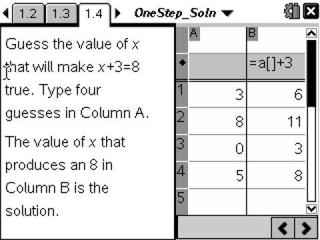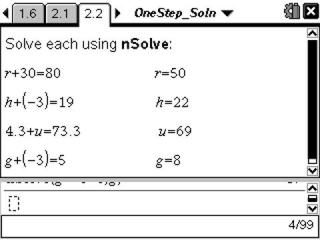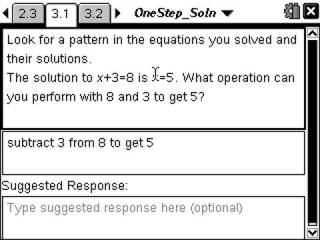# Activities

•##### Download

•• ##### Subject Area

• Math: Algebra I: Equations and Inequalities

• ##### Author9-12

45 Minutes

• ##### Device
• TI-Nspire™
• TI-Nspire™ CAS
• ##### Software

TI-Nspire™
TI-Nspire™ CAS

2.0

• ##### Report an Issue

One Step at a Time#### Activity Overview

Students solve one-step equations involving addition and multiplication by substituting possible values of a variable.

#### Key Steps

•Using a Spreadsheet application, students begin the activity by testing values for x in the equation x + 3 = 8, looking for the value of x that makes the equation true. They will practice find the solution with several equations that contain only addition.

•Then students use the nSolve command on the Calculator application to solve one step addition equations. By observing the solutions to many equations of the same form, students gather data to form a hypothesis about the solving an equation of this form. They will also form and solve their own equations to complete their understanding.

•Students will apply their hypothesis against the equations they solved on previous screens. Then they are introduced to the Subtraction Property of Equality.

At the end of the activity, students turn their attention to one-step equations involving operations other than addition. They will be introduced to the Division Property of Equality and the concept of inverse operations. Students will conclude by formulating a general rule for solving any one-step equation.